# VLOOKUP Function

The VLOOKUP function returns a value in the same row after finding a matching value in the first column.
XLOOKUP was added in Excel 2021 to replace this function.
This looks up a value in the first column of a table and returns a value in the same row but in a different column.
This function is not case sensitive.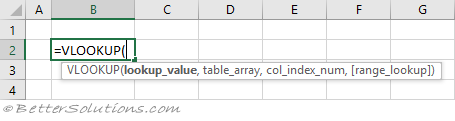### Return the Exact Match (unique values)

When the "range_lookup" argument is FALSE an exact match is found.
If there is more than one occurrence, the first occurrence is found.
The definition of the first occurrence is the same regardless of whether the table is sorted.
The table does not have to be sorted when you are looking for an exact match.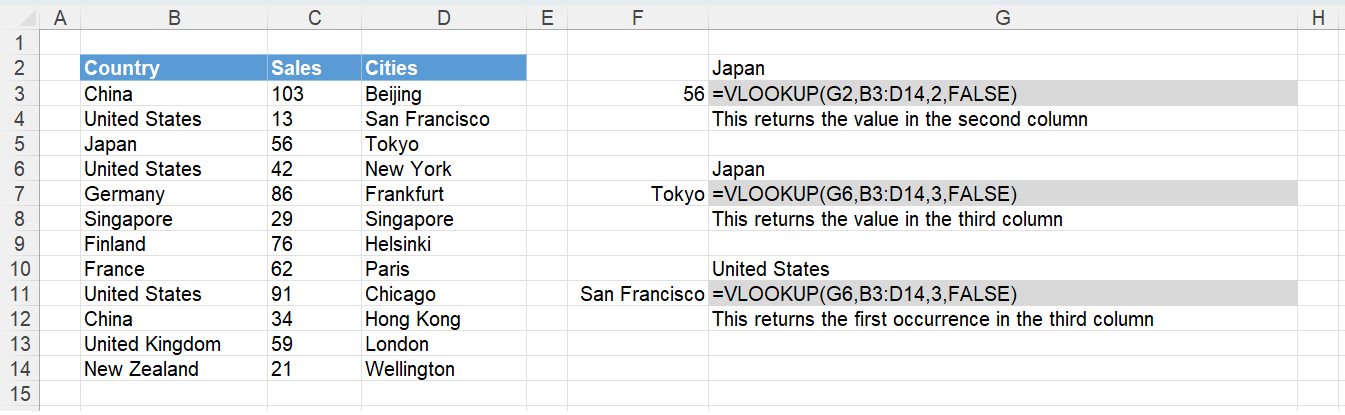### Return the Exact Match (item does not exist)

When the "lookup_value" is not in the list, #N/A is returned.
When the "range_lookup" argument is FALSE an exact match is found.
The table does not have to be sorted when looking for an exact match.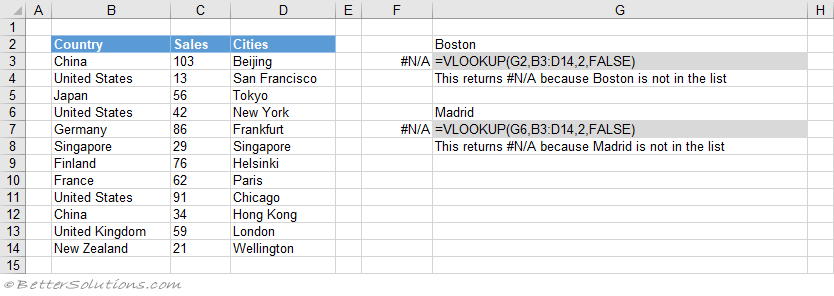### Return the Exact Match (case sensitive)

The VLOOKUP function is not case sensitive.
This means that upper case and lower case characters are equal (for example Japan = JAPAN).
You can perform a case sensitive lookup by using the INDEX, MATCH and EXACT functions.
The "0" passed to the MATCH function means that it will find an exact match.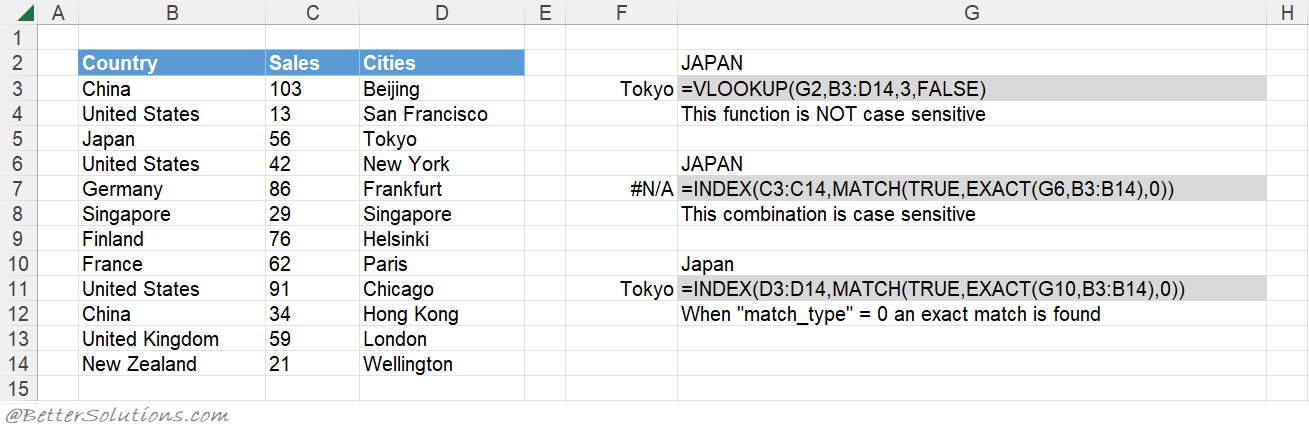### Return the Exact Match (first occurrence, sorted or not sorted)

This function will always return the first occurrence, starting at the top.
When the "range_lookup" argument is FALSE an exact match is found.
When the "range_lookup" argument is FALSE the first occurrence is always found.
The table does not have to be sorted to return the first occurrence.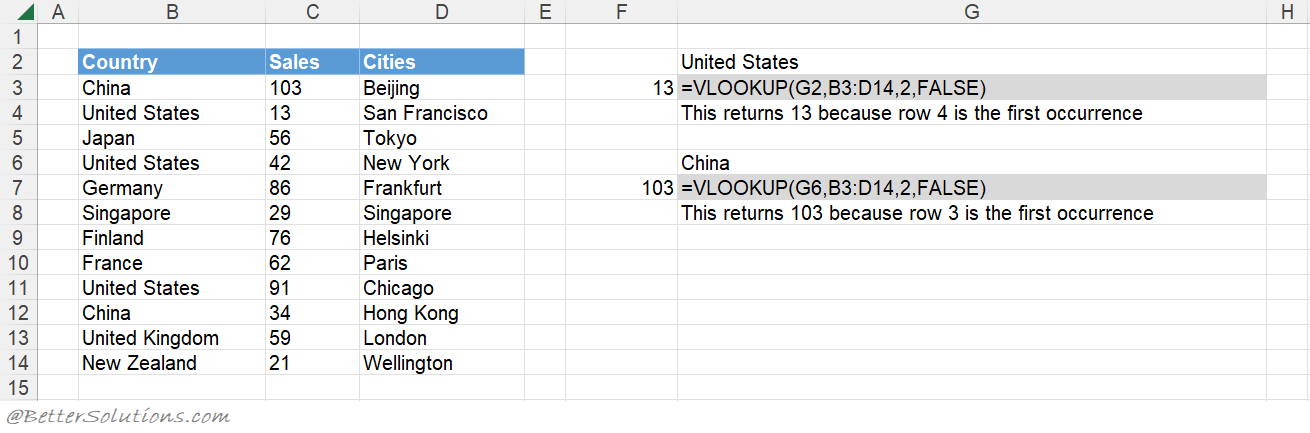### Return the Exact Match (last occurrence, sorted)

The last occurrence can be achieved when the "lookup_value" column is sorted into Ascending Order.
The "range_lookup" argument must be TRUE for the last occurrence to be used.
The table has been sorted by Sales and then by Country in ascending order.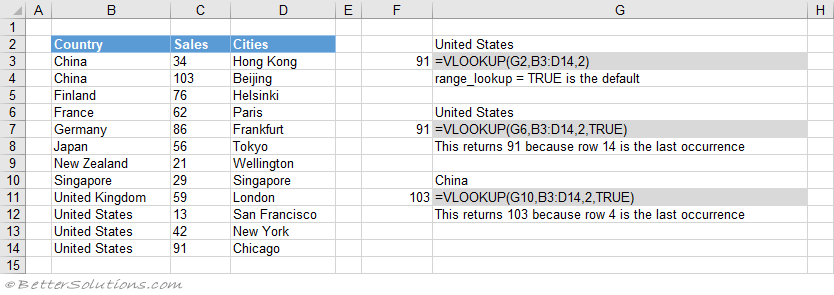### Return the Exact Match (last occurrence, not sorted)

You can get the last occurrence when the "lookup_value" column is not sorted by using the LOOKUP function.
The LOOKUP function uses an array of cell values and its closest match logic to find the last match.
The array eventually contains either 1's or error values and because the "lookup_value" is 2 it will return the position of the last 1 in the array.
You could use the INDEX and MATCH functions as an alternative.
You could use the INDEX, MAX and ROW functions as another alternative.
You could combine with the SUMPRODUCT function as another alternative.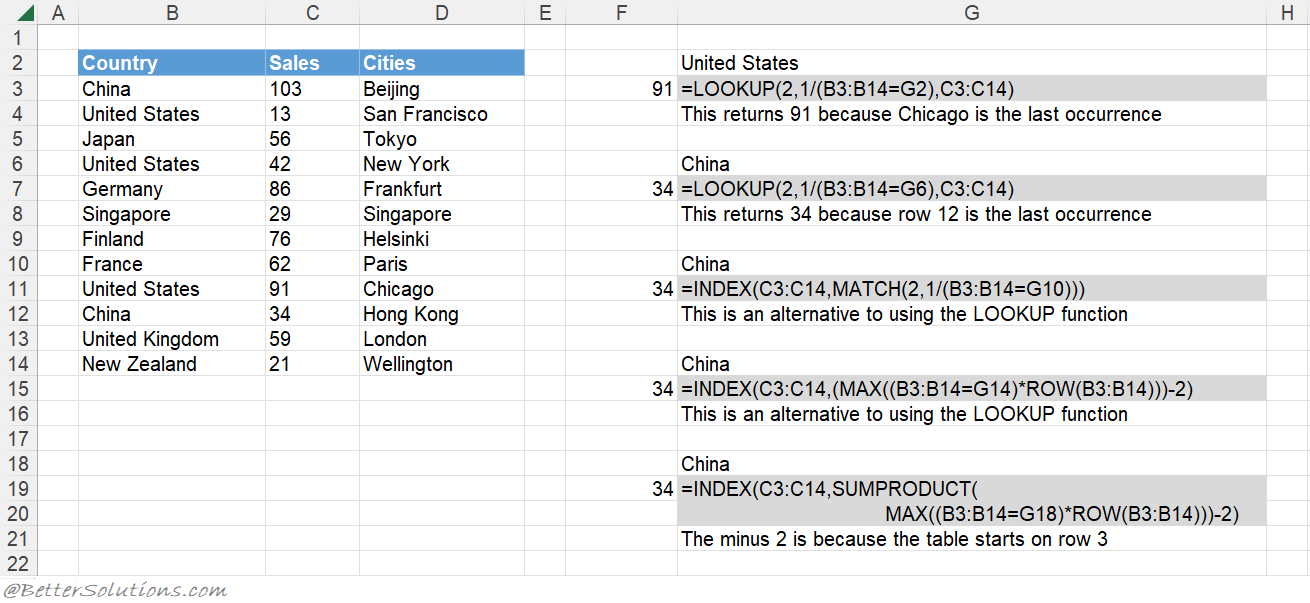### Return the Exact Match (with wildcard *)

An asterisk (*) matches any sequence of characters.
The "range_lookup" argument must be FALSE.
You can have an asterisk as the first character.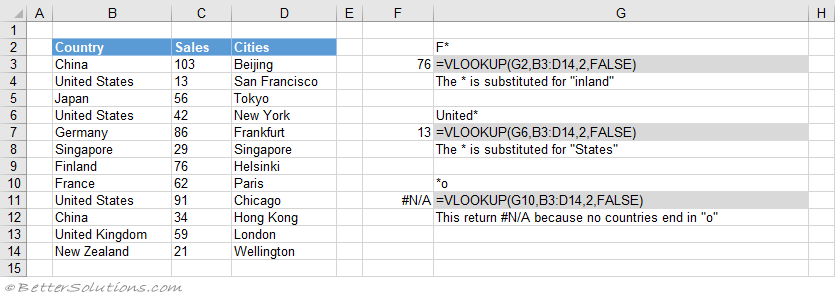### Return the Exact Match (with wildcard ?)

A question mark (?) matches any single character.
The "range_lookup" argument must be FALSE.
You can have a question mark as the first character.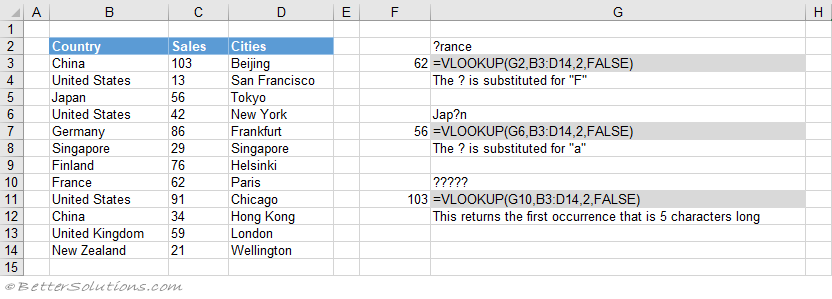### Return the Exact Match (multiple criteria)

When you want to return a value in the same row after finding matching values in 2 (or more) columns.

### Return the Closest Match (next smallest)

You can get the next smallest value when the "lookup_value" column is sorted into Ascending Order.
When the "range_lookup" argument is TRUE the nearest smallest value is found.
When the "range_lookup" argument is TRUE the table must be sorted.
The table has been sorted by Sales into ascending order.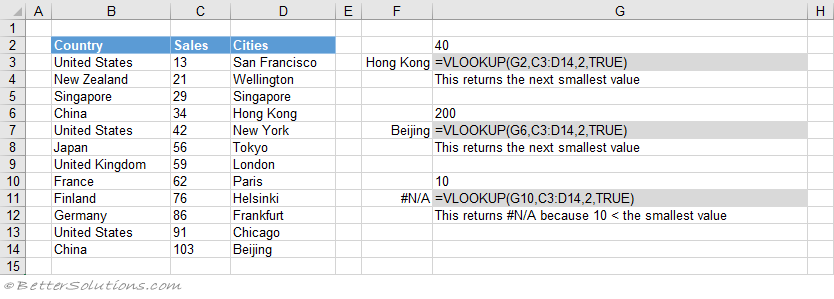### Return the Closest Match (next largest)

You can get the next largest value when the "lookup_value" column is sorted into Descending Order.
The next largest value can be returned by using the INDEX and MATCH combination.
The "-1" passed to the MATCH function means that it will find the smallest value that is greater than the lookup value.
The table has been sorted by Sales into descending order.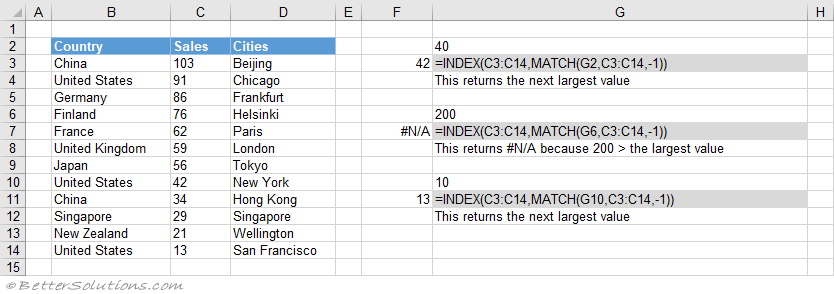### Combining with MATCH

A two way lookup can be achieved using a VLOOKUP and MATCH combination.
A two way lookup is when you return the intersection of a matching column with a matching row.

### Important

When no match is found and you want to return approximate values, the first column of your table must be sorted in ascending order.
If you are searching for numbers then they must be formatted as numbers and not as text. If they are formatted as text they will be ignored.
This function can only be used to return values in columns to the right of the lookup column.
The lookup column has to be the first column in the table.